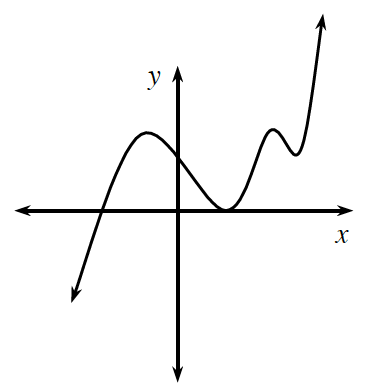### Home > CCA2 > Chapter Ch6 > Lesson 6.2.1 > Problem6-102

6-102.Consider the graph at right.

1. Is the graph a function? Explain.

What causes a graph to be a function or not a function?
Look up "function" in the glossary if you aren't sure.

2. Make a sketch of the inverse of this graph. Is the inverse a function? Justify your answer.

Reflect the graph across $y=x$.
Are there any x-values that have more than one y-value?

3. Must the inverse of a function be a function? Explain.

Consider several different functions and their inverses.

4. Describe what is characteristic about functions that do have inverse functions.

See part (a).
Think about how this rule is translated to the inverse.

5. Could the inverse of a non-function be a function? Explain or give an example.

Think about functions whose inverses are non-functions.
What about the inverse of that inverse?